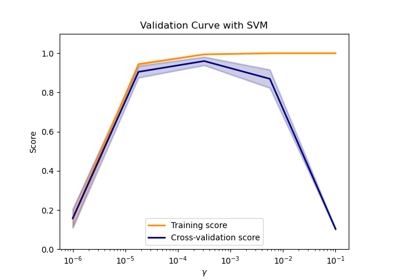# sklearn.model_selection.validation_curve¶

sklearn.model_selection.validation_curve(estimator, X, y, *, param_name, param_range, groups=None, cv=None, scoring=None, n_jobs=None, pre_dispatch='all', verbose=0, error_score=nan)[source]

Validation curve.

Determine training and test scores for varying parameter values.

Compute scores for an estimator with different values of a specified parameter. This is similar to grid search with one parameter. However, this will also compute training scores and is merely a utility for plotting the results.

Read more in the User Guide.

Parameters
estimatorobject type that implements the “fit” and “predict” methods

An object of that type which is cloned for each validation.

Xarray-like of shape (n_samples, n_features)

Training vector, where n_samples is the number of samples and n_features is the number of features.

yarray-like of shape (n_samples,) or (n_samples, n_outputs) or None

Target relative to X for classification or regression; None for unsupervised learning.

param_namestr

Name of the parameter that will be varied.

param_rangearray-like of shape (n_values,)

The values of the parameter that will be evaluated.

groupsarray-like of shape (n_samples,), default=None

Group labels for the samples used while splitting the dataset into train/test set. Only used in conjunction with a “Group” cv instance (e.g., GroupKFold).

cvint, cross-validation generator or an iterable, default=None

Determines the cross-validation splitting strategy. Possible inputs for cv are:

• None, to use the default 5-fold cross validation,

• int, to specify the number of folds in a (Stratified)KFold,

• An iterable yielding (train, test) splits as arrays of indices.

For int/None inputs, if the estimator is a classifier and y is either binary or multiclass, StratifiedKFold is used. In all other cases, KFold is used.

Refer User Guide for the various cross-validation strategies that can be used here.

Changed in version 0.22: cv default value if None changed from 3-fold to 5-fold.

scoringstr or callable, default=None

A str (see model evaluation documentation) or a scorer callable object / function with signature scorer(estimator, X, y).

n_jobsint, default=None

Number of jobs to run in parallel. None means 1 unless in a joblib.parallel_backend context. -1 means using all processors. See Glossary for more details.

pre_dispatchint or str, default=’all’

Number of predispatched jobs for parallel execution (default is all). The option can reduce the allocated memory. The str can be an expression like ‘2*n_jobs’.

verboseint, default=0

Controls the verbosity: the higher, the more messages.

error_score‘raise’ or numeric, default=np.nan

Value to assign to the score if an error occurs in estimator fitting. If set to ‘raise’, the error is raised. If a numeric value is given, FitFailedWarning is raised. This parameter does not affect the refit step, which will always raise the error.

New in version 0.20.

Returns
train_scoresarray of shape (n_ticks, n_cv_folds)

Scores on training sets.

test_scoresarray of shape (n_ticks, n_cv_folds)

Scores on test set.

Notes

## Examples using sklearn.model_selection.validation_curve¶